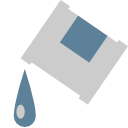# Fill nodeThis documentation is for version 1.0 of Fill (fr.inria.Fill).

## Description

Add a constant color on the source image where the alpha channel not 0. You can control the blending between the original image and the constant color with the operator and the mix factor.

Input

Description

Optional

Source

No

Yes

## Controls

Parameter / script name

Type

Default

Function

Convert to Group / `convertToGroup`

Button

Converts this node to a Group: the internal node-graph and the user parameters will become editable

Color / `Solid1color`

Color

r: 0 g: 0 b: 0

Operation / `Merge1operation`

Choice

over

atop: Ab + B(1 - a) (a.k.a. src-atop)
average: (A + B) / 2
color: SetLum(A, Lum(B))
color-burn: darken B towards A
color-dodge: brighten B towards A
conjoint-over: A + B(1-a)/b, A if a > b
copy: A (a.k.a. src)
difference: abs(A-B) (a.k.a. absminus)
disjoint-over: A+B(1-a)/b, A+B if a+b < 1
divide: A/B, 0 if A < 0 and B < 0
exclusion: A+B-2AB
freeze: 1-sqrt(1-A)/B
from: B-A (a.k.a. subtract)
geometric: 2AB/(A+B)
grain-extract: B - A + 0.5
grain-merge: B + A - 0.5
hard-light: multiply(2*A, B) if A < 0.5, screen(2*A - 1, B) if A > 0.5
hue: SetLum(SetSat(A, Sat(B)), Lum(B))
hypot: sqrt(A*A+B*B)
in: Ab (a.k.a. src-in)
luminosity: SetLum(B, Lum(A))
matte: Aa + B(1-a) (unpremultiplied over)
max: max(A, B) (a.k.a. lighten only)
min: min(A, B) (a.k.a. darken only)
minus: A-B
multiply: AB, A if A < 0 and B < 0
out: A(1-b) (a.k.a. src-out)
over: A+B(1-a) (a.k.a. src-over)
overlay: multiply(A, 2*B) if B < 0.5, screen(A, 2*B - 1) if B > 0.5
pinlight: if B >= 0.5 then max(A, 2*B - 1), min(A, B * 2) else
reflect: A*A / (1 - B)
saturation: SetLum(SetSat(B, Sat(A)), Lum(B))
screen: A+B-AB if A or B <= 1, otherwise max(A, B)
soft-light: burn-in if A < 0.5, lighten if A > 0.5
stencil: B(1-a) (a.k.a. dst-out)
under: A(1-b)+B (a.k.a. dst-over)
xor: A(1-b)+B(1-a)

Mask / `Merge1enableMask_Mask`

Boolean

Off

/ `Merge1maskChannel_Mask`

Choice

None

Invert Mask / `Merge1maskInvert`

Boolean

Off

Mix / `Merge1mix`

Double

1